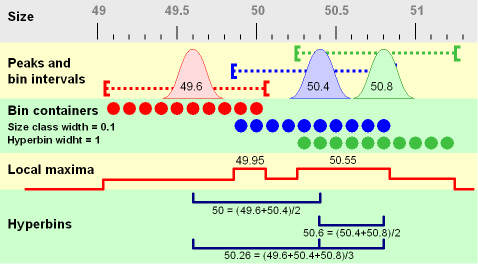Hyperbinning Details - Examples Previous Top Next

Because the sizing of fragments yields non-integer length values (floating values) we can imagine two samples with the following fragment lengths:

 Example 1 Sample 1: Peak at 49.6 bp Sample 2: Peak at 50.4 bp Can we throw these two peaks into a common bin? Yes, if we have a bin with the label '50' on it, both peaks fit into this bin. In other words: both samples have a fragment with a length of about 50 base pairs. Note: The length difference between both sample peaks is: 50.4 - 49.6 = 0.8. So both peaks are about 0.8 bases apart. The mean fragment length is 50 and the (hyper)bin covers fragments with a size from from 49.5 to smaller than 50.5.Example 2 Peak #1: Peak at 49.6 bp Peak #2: Peak at 50.4 bp Peak #3: Peak at 50.8 bp The fragments of sample #1 and #2 (peaks #1 and #2) fit into a single hyperbin (total fragment length difference = 0.8, i.e. smaller than 1). The fragments of sample #2 and #3 also fit into a hyperbin (total fragment length difference = 0.4, i.e. smaller than 1).. However, a problem arises if all three samples are compared. Then the total fragment length difference would be 50.8-49.6 = 1.2. In practice the SequentiX maximum detector generates size classes comprising fragments with (length >= size class - 0.5) AND (length < size class + 0.5) . The local maximum detector detects a maximum in the range of 49.9 to 50.0 (with its arithmetic mean at 49.95) to which peak #1 and peak #2 contribute. A second maximum is detected from 50.3 to 50.8 (mean = 50.55). The mean values of the maxima are separated by 0.6 units. Please note that peak #2 contributes to both local maxima.Is there an affinity of peak #2 to one of the two other peaks? There is a larger overlap between peak #2 and peak #3. However, if we would analyse sample #1 and #2 only (see example 1 above) peak #2 and peak #1 should belong to the same hyperbin because their length difference is 0.8, i.e. smaller than 1. The smallest length difference that can be found between two DNA fragments is 1, meaning that one fragment is a minimum of one base longer than another one. This is the reason why we put the above detected local maxima (length difference = 0.6) into a single common container. This hyperbin comprises all three peaks and covers a length difference of 1.2 bases which is illogical in a biological sense but has been explained in the above examples.